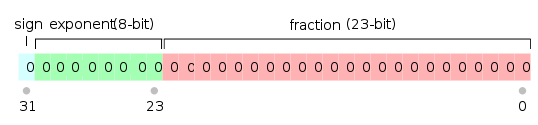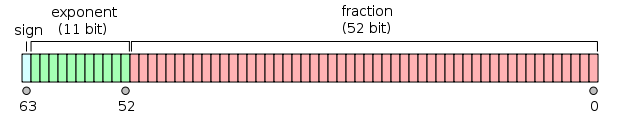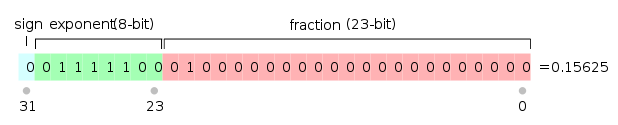# 【算法】解析IEEE 754 标准

contents structure [-]

IEEE 754(Institute of Electrical and Electronics Engineers)在1985年发布,该标准是为了统一规范浮点数的存储。

# 1.浮点数的存储过程

1个符号位
8个指数位
23个小数位1个符号位
11个指数位
52个小数位0.1562510转化为二进制就是0.001012，然后将该数写成科学计数法（scientific notation），根据IEEE 754的规定，小数点的左边只能有一个1，所以最终的科学计数法形式是：

0.1562510 = 0.001012 = 1.012 * 2-30 == 特殊情况：零（zero） 或 次正规数（subnormal）
1 == 2 ^ -126
...
125 == 2 ^ -2
126 == 2 ^ -1
127 == 2 ^  0
128 == 2 ^  1
129 == 2 ^  2
...
254 == 2 ^ 127
255 == 特殊情况：无穷大（infinity） 或 非数值（NaN）

## 1.1 次正规数（Denormalized Number）

IEEE 754的设计者注意到，除了0.0所有的二进制的科学计数法都有一个1在小数点的左边。在上面也提到过，在写成标准的科学计数法的形式后，小数点的左边只能有一个1。

25.010 == 110012 = 1.10012 * 24
0.62510 == 0.1012 = 1.012 * 2-1

0 00000001 000000000000000000000012 = 1.000000000000000000000012 * 2-126

0 00000000 000000000000000000000012 = 0.000000000000000000000012 * 2-126

## 1.2 零（zero）

符号位（sign） = 0或1

0的内存二进制码为：

0 00000000 00000000000000000000002
1 00000000 00000000000000000000002

## 1.3 非数值（NaN）

符号位（sign） = 0或1

0 11111111 111111111111000000100002

1 11111111 111111111111000000100002

## 1.4 无穷大（infinity）

符号位（sign） = 0表示正无穷大，1表示负无穷大。

0 11111111 000000000000000000000002

1 11111111 000000000000000000000002

# 2.除数为0.0会发生什么

#include <iostream>
#include <limits>
int main(){
//is_iec559是否支持IEC-559 / IEEE-754标准
std::cout << std::numeric_limits<float>::is_iec559 << std::endl;
std::cout << (1.0 / 0.0) << std::endl;
std::cout << (-1.0 / 0.0) << std::endl;
std::cout << (0.0 / 0.0) << std::endl;
return 0;
}

1
inf
-inf
-nan

# 3.浮点数的范围

0 00000000 000000000000000000000012 = 0000 000116 = 0.12 × 2-22 × 2-126  =  2−126 × 2−23 = 2−149 ≈ 1.4012984643× 10−45

（最小的次正规数，smallest positive subnormal number）

0 00000000 111111111111111111111112 = 007f ffff16 = 0.111111111111111111111112 * 2-126  =  2−126 × (1 − 2−23) ≈ 1.1754942107 ×10−38

（最大的次正规数，largest subnormal number）

0 00000001 000000000000000000000002 = 0080 000016 = 1.02 × 21-127 = 2−126 ≈ 1.1754943508 × 10−38

（最小的正正规数，smallest positive normal number）

0 11111110 111111111111111111111112 = 7f7f ffff16 = 1.111111111111111111111112  × 2254-127 =  2127 × (2− 2−23) ≈ 3.4028234664 × 1038

（最大的正正规数，largest normal number）

0 01111110 111111111111111111111112 = 3f7f ffff16 = 1.111111111111111111111112 × 2126-127 = 1 − 2−24 ≈ 0.9999999404

（比数值1小的最大数，largest number less than one）

0 01111111 000000000000000000000002 = 3f80 000016 = 1.02 × 2127-127  = 1.02 × 20 = 1

（数值1，one）

0 01111111 000000000000000000000012 = 3f80 000116 = 1.000000000000000000000012 × 2127-127 = 1 + 2−23 ≈ 1.0000001192

（比数值1大的最小数，smallest number larger than one）

1 10000000 000000000000000000000002 = c000 000016 = −2

0 00000000 000000000000000000000002 = 0000 000016 = 0

1 00000000 000000000000000000000002 = 8000 000016 = −0

0 11111111 000000000000000000000002 = 7f80 000016 = infinity（正无穷）

1 11111111 000000000000000000000002 = ff80 000016 = −infinity（负无穷）

0 10000000 100100100001111110110112 = 4049 0fdb16 ≈ 3.14159274101 ≈ π （ 圆周率，pi ）

0 01111101 010101010101010101010112 = 3eaa aaab16 ≈ 0.333333343267 ≈ 1/3

x 11111111 100000000000000000000012 = ffc0 000116 = qNaN (on x86 and ARM processors)

x 11111111 000000000000000000000012 = ff80 000116 = sNaN (on x86 and ARM processors)

 Level Width Range at full precision Single precision 32bits ±1.18×10−38 to ±3.4×1038 Double precision 64 bits ±2.23×10−308 to ±1.80×10308

# 4.浮点数的精度

10n = 223
10n = 8388608
106 < 8388608 < 107

#include <stdio.h>      /* printf */
#include <math.h>       /* fabs */

int main ()
{
float f1 = 0.007;
float f2 = 0.009;

int res = ( fabs(f1-f2) < 1e-6 );
printf ("f1 == f2 is : %s\n",res?"true":"false");
return 0;
}

f1 == f2 is : false

# 5.参考文献

posted @ 2019-03-10 17:33  HDWK  阅读(13008)  评论(2编辑  收藏  举报